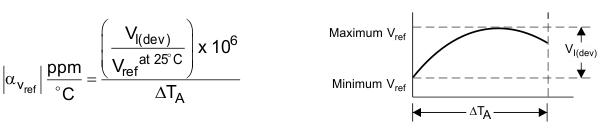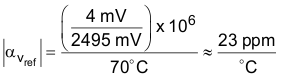JAJSHO6F March   2005  – July 2019

PRODUCTION DATA.

1. 特長
2. アプリケーション
3. 概要
1.     Device Images
4. 改訂履歴
5. Pin Configuration and Functions
6. Specifications
7. Parameter Measurement Information
8. Detailed Description
9. Application and Implementation
1. 9.1 Application Information
2. 9.2 Typical Applications
1. 9.2.1 Comparator Application
2. 9.2.2 Other Application Circuits
10. 10Power Supply Recommendations
11. 11Layout
12. 12デバイスおよびドキュメントのサポート
13. 13メカニカル、パッケージ、および注文情報

#### パッケージ・オプション

デバイスごとのパッケージ図は、PDF版データシートをご参照ください。

• DBZ|3
• DBV|5

#### 9.2.1.2.5 Deviation Parameters and Calculating Dynamic Impedance

The deviation parameters, VI(DEV) and II(DEV), are defined as the differences between the maximum and minimum values obtained over the recommended temperature range. The average full-range temperature coefficient of the reference voltage (αVref) is defined in Equation 1.

Equation 1.where

• ΔTA is the recommended operating free-air temperature range of the device

αVref can be positive or negative, depending on whether minimum VREF or maximum VREF, respectively, occurs at the lower temperature.

Example:

Maximum VREF = 2496 mV at 30°C, minimum VREF = 2492 mV at 0°C, VREF = 2495 mV at 25°C, ΔTA = 70°C for TL43x.

Equation 2.Because minimum VREF occurs at the lower temperature, the coefficient is positive.

The dynamic impedance is defined as |ZKA| = ΔVKA / ΔIKA.

When the device is operating with two external resistors, see Figure 21, the total dynamic impedance of the circuit is given by Equation 3.

Equation 3.